International Journal of Energy and Power Engineering
Volume 5, Issue 2, April 2016, Pages: 83-89

Numerical Analysis of Thermosyphon Solar Water Heaters

Samuel Sami, Edwin Marin, Jorge Rivera

Research Center for Renewable Energy, Catholic University of Cuenca, Cuenca, Ecuador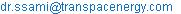(S. Sami)

Samuel Sami, Edwin Marin, Jorge Rivera. Numerical Analysis of Thermosyphon Solar Water Heaters. International Journal of Energy and Power Engineering. Vol. 5, No. 2, 2016, pp. 83-89. doi: 10.11648/j.ijepe.20160502.18

Received: January 26, 2016; Accepted: February 3, 2016; Published: May 11, 2016

Abstract: This paper presents the modeling and simulation as well as validation of a natural circulation closed thermosyphon glass tube solar collector water heater. Energy conservation equations for the heat transfer fluid flow and the storage tank were written in finite-difference form, integrated and solved to yield the characteristics of the thermosyphon system at different solar insolations and water mass flow rate conditions as well as water temperatures. Comparison between experimental data and numerical prediction of the proposed showed that the model predicted fairly the evacuation of storage tank temperature at various initial temperature of the water at the storage tank.

Keywords: Thermal Solar Collector, Thermosyphon, Water Heater, Modeling, Analysis Validation

Contents

1. Introduction

Water heating typically represents a significant percentage of energy consumption in domestic, industrial applications. Carbon baseline fuels water heating produce emissions of greenhouse gases and other pollutants. Due to high cost primary energy resources and their associated with serious environmental issues, solar energy is an alternative viable source of energy for water heating. Solar water heating can be characterized as active or passive [1-5]. An active system is based on an electric pump to circulate the working fluid through the solar collector. In passive solar water heating, heat-transfer fluid uses thermosyphoning phenomenon to circulate the water by the buoyancy forces and is replaced by colder water from the bottom of the tank . This continuous circulation continues until heats up water in the storage tank (Figure 1).

Abgo reported on the performance profile of a thermosyphon solar water heater . The performance evaluation was based on the mathematical models that describe the test system and some measured experimental data. The effect of some of the design and operating parameters that have been shown to affect the system’s performance was investigated. Numerical simulation of steady state natural convection heat transfer in a 3-dimensional single-ended tube subjected to a nanofluid has been presented by Shahi et al. . It was a simplified model for single-ended evacuated solar tube of water glass evacuated solar water tube heater. It was assumed in the model that the water sealed tube is adiabatic and also the tube opening is subject to copper-water nanofluid. Governing equations were based upon cylindrical coordinate system.

Another numerical analysis of a modified evacuated tubes solar collector was presented by Sato, et al. . This paper proposed a study of solar water heating with evacuated tubes, their operation, characteristics and operating parameters. Furthermore, another analytical study was reported by Hammadi  on the study of solar water heating system with natural circulation in Basrah. The results show that the performance of the solar water heater depends on parameters such as tilt angle and orientation of the collector, wind velocity, area of the collector, latitude, and solar time.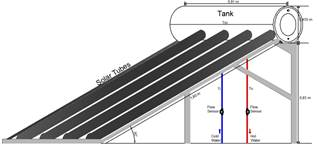Figure 1. Thermosyphon solar water heating system.

2. Mathematical Model

A schematic of the system under study is depicted in Figure 1. The system consists of a thermal solar panel, with solar glass tubes as water tube heater and control valves. Specifications of the solar glass tube collector are given in Table.1. As in passive solar water heating system, the water as heat-transfer fluid uses thermosyphoning phenomenon to circulate between the solar tubes and tank. With the buoyancy forces colder water is replaced from the bottom of the tank. This continuous circulation continues until heats up water in the storage tank (Figure 1). The storage was filled with 150 liters of water and the working fluid tubes were filled with working fluid. The working fluid flows inside the tubes in the collectors and by thermosyphoning back to the solar water storage tank. In the solar collector the solar radiation is absorbed by the working fluid. Due to the absorption of solar radiation, working fluid temperature increases. Thus, because of the difference of working fluid densities, the heated working fluid moves upward to the storage tank. The heat from the working fluid is transferred to the cold water and increases its temperature in storage tank. The following parameters were recorded at 1 minute intervals for the purpose of validation of the proposed model; cold water temperature working fluid temperature, solar collector temperature, storage tank temperature, solar insolation (W/m2), ambient temperature, wind velocity (m/sec.), hot water temperature and relative humidity (%).

The conservation equations and heat transfer equations were written for each water solar glass tube collector, storage tank and heat transfer fluid as follows;

Energy conservation and heat transfer equations:

The mass flow rate circulating through the solar glass tube collector can be calculated using the following formula ;(1)

Equation (1) can be rewritten as follows;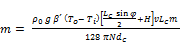(2)

and;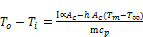(3)

Where Tm is;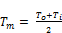(4)

The heat transfer coefficient of wind is given by [9,10];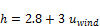(5)

In addition, the following parameters; density, specific heat, coefficient of water expansion, as well as volume of storage tank are defined as follows respectively;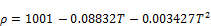(6)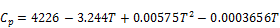(7)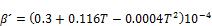(8)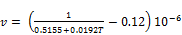(9)

Meanwhile, the energy equation for the thermosyphon solar water heater during daily solar radiation can be written as follows ;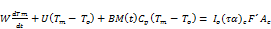(10)

The rate of water evacuated from the storage tank is given by;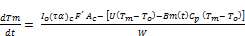(11)

Where τα_ is effective transmittance-absorptance and F1 represents collector efficiency factor, and U is collector overall heat loss coefficient.

W, and Tm are the total heat capacity of the system, and the mean system temperature respectively. B is a constant which can be taken as 1 if water is drawn from the middle of the tank and 2 if it drawn from the top of the tank .

The solar collector energy conversion efficiency can be calculated as follows;

Ƞc= Qu/Ac Io                                    (12)

Where Qu is energy absorbed by the collector.

The energy absorbed by the collector can be obtained as follows ;

Qu = Ac F1[ Io (τα) – Ul ( Tm-Ta)]                  (13)

Where,

F1: is the collector efficiency factor

Ul: is the collector overall heat loss coefficient (W/m2K)

Solving the aforementioned mentioned equations yield the water mass flow rate evacuated from the storage tank, energy absorbed by solar collector, energy conversion efficiency as well the storage tank temperature during the water evacuation from the tank.

3. Numerical Procedure

The energy conversion and heat transfer mechanisms taking place during the thermosyphoning process in a thermal solar panel glass tubes have been outlined in equations (1) through (13). The aforementioned equations have been written in finite-difference form, solved as per the logical flow diagram shown in Figure 2 as a function of time. First the input independent parameters are defined and other dependent parameters were calculated and integrated to yield evacuated water mass flow rate as per equation (2) and the temperature gradient in the storage tank as per equation (11). Iterations were performed until a solution is reached with acceptable iteration error. The numerical procedure starts with using the solar radiation to calculate the mass flow water circulating in the solar panel due buoyancy forces. This follows by predicting the water temperature profile as a function of time.

Table 1. Specifications of the water glass solar tubes collector.

 SUNSHORE VACUUM TUBES SPECIFICATION Model 58*1800 Material Borosilicate glass Outer tube dia Ȼ 58 mm Inner tube dia Ȼ 47 mm Tubes length 1800 mm Tubes number 10 Thickness 1.6 mm Vacuum inner pressure ≤ 5.0 x 10-3 Pa Absorptivity ≥ 0.92 (AM 1.5) Emissivity ≤ 0.08 (80°C ± 5°C) Absorptivity coating Al/Copper/Stainless or Al/N/AL Thermal expansion 3.3 Stagnation temperature ≥ 270°C Heat loss 22 W/(m3, K) Maximum strength 30mm iron ball dropped directly against the tubes from 450mm high attitude, the tube without any damages Anti-freezing - 30°C Resist Hailstone 25 mm diameter hailstone Life time 15 years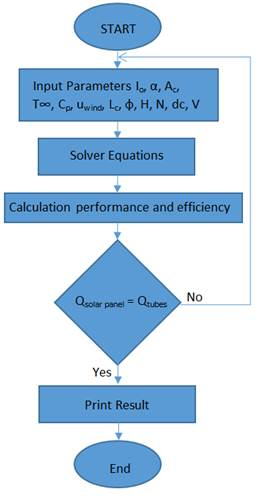Figure 2. Logical flow diagram.

4. Results and Discussion

The thermosyphon solar water heater shown in Figure. 1, has specifications outlined in Table. 1. The storage tank capacity of 150 liters was connected to plastic pipes connections for the circulation of the water from and to the collector. In this section, we first present numerical simulation results of the solar water heater system presented in reference  under various conditions and secondly validate our proposed model with experimental data.

The aforementioned system of equations (1) through (13) in finite-difference formulation has been numerically solved and samples of the predicted results are plotted in Figures 3 through 15 under different inlet conditions such as insolation, heat transfer fluid flow rates and heat transfer fluid temperatures as well as solar panel inclination angles. In particular, Figure. 3 presents the daily time variation of solar insolation (W/m2) measured at the site and employed in the validation of the proposed model. It is quite clear that the intensity of radiations depends upon the hour of the day and the month of the year. The average values of radiation insolation were used in the present study.

In general, it is quite clear from these figures (4) through (11) that temperature of water in the storage tank decreases during the evacuation process and the rate of temperature drop has functional dependence on the various parameters outlined in equations (1) through (13). In the following sections, the simulation results of the storage tank temperature will be presented and analyzed under different conditions.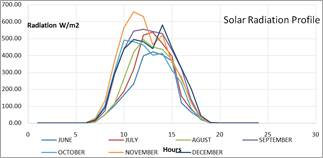Figure 3. Time variation of solar insolation during during 2015.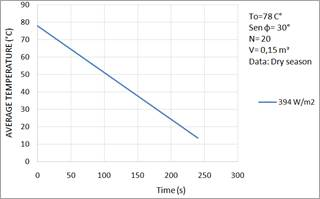Figure 4. Time variation of initial water temperature in storage tank.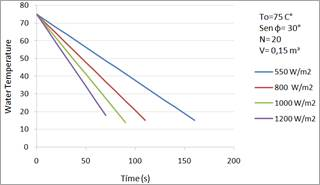Figure 5. Time variation of initial water temperature in storage tank at various solar radiations.

The simulated results presented in Figures. 5 through. 11 show that the water temperature in the storage tank is principally a function of solar radiation and the ambient air conditions. However, a typical analysis of the simulated results presented in Figures 7 through 11 also shows that the water temperature in the storage tank depends upon other parameters such as collector orientation, number of pipes in the solar collector and tank volume, the rate of evacuation of the water from the tank and the makeup water.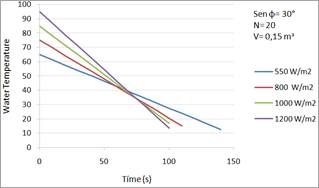Figure 6. Time variation of different initial water temperature in storage tank at different solar radiations.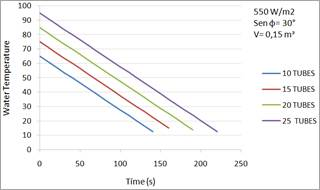Figure 7. Time variation of different initial water temperature in storage tank with various heat tubes in solar collector.

In particular, Figure. 5 shows that impact of solar intensity on the rate of decrease temperature inside the storage tank. It can be also observed from this figure that the lower the radiation insolation is the slower rate of temperature reduction.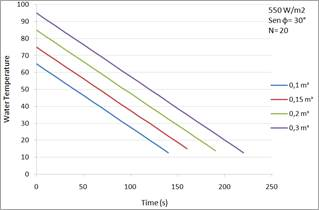Figure 8. Time variation of initial water temperatures in different storage tank volumes.

The simulation results presented in Figure. 6 illustrate the impact of initial storage tank temperature on the rate of decrease of temperature in the storage tank under different solar insolation at constant water evacuation flow rate from the tank. It is quite clear from this figure that longer period for water evacuation occurs at lower solar insolation and lower initial storage tank temperature.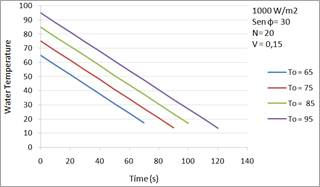Figure 9. Time variation of initial water temperature in storage tank at different outlet water temperatures.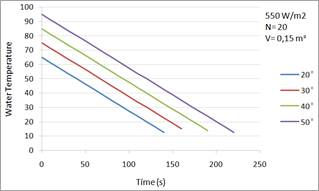Figure 10. Time variation of initial water temperature in storage tank at different solar tubes inclination angles.

However, Figure. 7 showed that increasing the number of solar tubes will prolong the water evacuation time of the storage water tank at constant solar insolation. It is also evident from the results presented in this figure that the higher the number of solar tubes the higher the water storage tank temperature.

Figure 8 has been constructed to examine the impact of the storage tank volume on the time variation of initial water temperature and the water evacuation time from the storage tank. It is obvious that the bigger the storage tank volume the longer period the evacuation takes.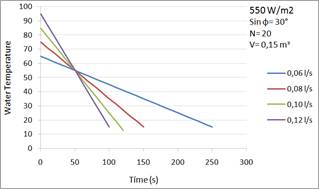Figure 11. Time variation of initial water temperature in storage tank at various evacuation flow rates.

It was also observed from the simulation results that the initial maximum storage tank temperature exhibits significant influence on the evacuation time, therefore, this figure is significant in presenting the impact of the initial temperature in the storage tank. The figure also shows that the higher the initial temperature the longer the evacuation period.

On the other hand, Figure 10 presents the effect of changing the inclination angle of the solar tubes collector. It appears from this figure that the bigger the angle the longer is the evacuation period. Furthermore, Figure. 11 has been presented to illustrate the impact of water evacuation flow rates at constant solar insolation on the evacuation period. As shown in this figure, the higher evacuation flow rate is the quicker the evacuation time. It is worthwhile mentioning that the evacuation flow rate depends upon the solar water heater application. Normally, this type of solar water heater is equipped with a control system panel and temperature setting controller and a temperature sensor inside the tank as well as an electric heater to ensure that the desired temperature is always supplied. This electrical heater can be used when solar intensity is very low or at extreme cold water conditions to ensure the desired temperature is supplied.

In order to validate the prediction of the proposed model presented in the aforementioned equations (1) through (13), Figures 12 through 14 have been constructed to simulate the data presented in these figures. The predicted results were based upon the following experimental set up for the water glass solar tubes [(Table. 1) and Figure. 1]; inclination angle of 30° (Sin φ= 30°), number of solar tubes = 10, and V= 0.15 mᵌ. The flow of water leaving the tank was kept constant during the simulations presented in these figures. The temperatures were measured with sensors Tip 3-pin transistor DS18B20 with precision ±0.5°C, and analog multi meter EM5510 with resolution 0.1°C and resolution accuracy ± 1.5% ± 3°C.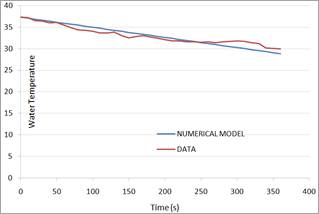Figure 12. Comparison between model prediction and experimental data.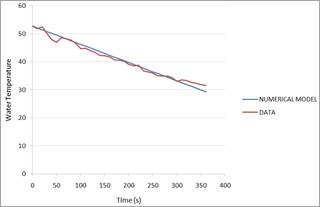Figure 13. Comparison between model prediction and experimental data.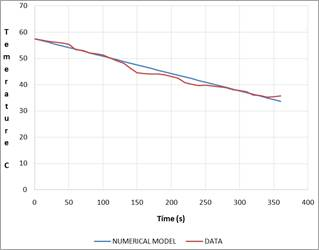Figure 14. Comparison between model prediction and experimental data.

The water flowrates were measured with flow meters type Hall effect model number YFG1 with precision +/- 3% of the flow rate in the range of 1-10 l/min. The solar intensity was measured at the site using a HOBO ware software package for HOBO data loggers.

It is quite clear from these figures that the water temperature in the storage tank decreases as water is evacuated from the tank and makeup city water is supplied to the storage tank. It is also evident from the comparison presented in these figures that the proposed model predicted fairly the evacuation storage tank time variation temperature at various initial temperatures of the water at the storage tank and solar radiations as measured by the Hobo data acquisition system. It can also be pointed out that the results presented in these figures show minor discrepancies between the predicted results and data which are attributed to heat losses. The higher the storage tank temperature the higher the heat losses.

The energy conversion efficiencies of the solar collector were calculated using equations (12) and (13) and presented in figure. 15, for various solar radiations and initial water temperatures in the storage tank. The results illustrated, in this figure, clearly show that higher solar radiation enhances the solar collector energy conversion efficiency. Also as shown, lower water temperature in the storage tank is associated with higher efficiency. This is attributed to less heat losses from the storage tank during the water heating process.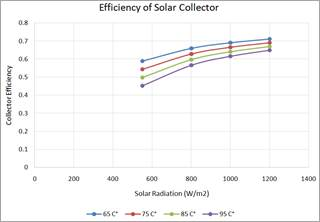Figure 15. Energy conversion efficiency of solar collector.

5. Conclusions

During the course of this study, modeling and simulation as well as validation of a natural circulation closed thermosyphon glass solar tube collector water heater have been presented and discussed. Energy conservation equations were written for heat transfer fluid flow from the storage, in finite-difference form, integrated and solved to yield the behavior of the thermosyphon system at different solar insolations and water mass flow rate conditions as well as water storage tank temperatures.

The results presented in this study showed that higher solar radiation enhances the solar collector energy conversion efficiency. It is quite evident from the comparison presented in hereby that the proposed model predicted fairly the evacuation storage tank time variation temperature at various initial conditions of the water at the storage tank and solar radiations.

Acknowledgement

The research work presented in this paper was made possible through the support of the Catholic University of Cuenca.

Nomenclature

 A Collector area (m2) d Diameter of glass solar tube g Acceleration due to gravity (m/s2) H Height of tank (m) I Solar intensity (W/m2) L Length of the collector (m) m Water mass flow rate (kg/s) N Number of tubes in the collector (–) u Velocity (m/s) T Temperature (°C) t Time (hr) Greek symbols a Absorbitivity (-) τα Effective transmittance-absorptance ρ Water density (kg /m3) β´ Coefficient of expansion of water (k-1) ʋ Mean kinematics viscosity of water (m2/s) Indices c Collector s Storage tank o or i Outlet and Inlet of the collector, respectively

References

1. Mahendra S. Seveda, "Performance analysis of solar water heater in NEH region of India", International Journal of Renewable and Sustainable Energy, 2013; 2 (3): 93-98.
2. O. B. Bukola, "Flow design and collector performance of a natural circulation solar water heater" Journal of Engineering and Applied Science, Vol. 1, Issue: 1, pp. 7-13, 2006.
3. B. Sitzmann, "Solar Water Heater with Thermosyphon Circulation", Appropriate Technology, Vol. 31, Issue: 1, pp. 66-70, 2004.
4. D. J. Close, "The Performance of Solar Water Heaters with Natural Circulation", Solar Energy, Vol. 6, Issue: 1, pp. 33-40, 1962.
5. J. Huang, S. Pu, W. Gao, and Y. Que, "Experimental investigation on thermal performance of thermosyphon flat-plate solar water heater with a mantle heat exchanger", Energy, Vol. 35, pp.3563-3568, 2010.
6. S. Abgo "Analysis of the performance profile of the NCERD thermosyphon solar water heater", Journal of Energy in Southern Africa, Vol 22 No 2, May 2011.
7. M. Shahi, A. Mahamoudi, and F. Talebi, "Numerical simulation of steady natural convection heat transfer in a 3-dimensional single-ended tube subjected to nanofluid", International Communications in Heat and mass Transfer, 37, pp. 1535-1545, 2010.
8. A. I. Sato, V. L. Scalon and A. Padilha, "Numerical analysis of a modified evacuated tubes solar collector" International Conference on Renewable Energies and Power Quality (ICREPQ’12), Santiago de Compostela (Spain), 28th to 30th March, 2012.
9. S.Hammadi, "Sudy of solar water heating system with natural circulation in Basrah" Al-Qadisi Journal for Engineering Sciences Vol. 2, No.3, 2009.
10. T.T. Chow, W. He, and J.Ji, "Hybrid photovoltaic thermosyphon water heating system for residential application" J. Solar Energy, Vol., 80, pp.298-306, 2006.

 Contents 1. 2. 3. 4. 5.
Article ToolsAbstractPDF(1715K)